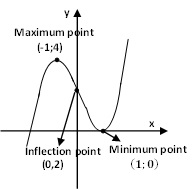# Sketch the following curve, indicating all positive extreme points and inflection points y=x^3-3x+2

## Question:

Sketch the following curve, indicating all positive extreme points and inflection points {eq}y=x^3-3x+2 {/eq}

## Concavity:

To calculate the intervals the concavity and convexity of a function we can follow the following steps:

1. Find the second derivative and calculate its roots.

2. We form open intervals with the zeros (roots) of the second derivative and the points of discontinuity (if any).

3. We take a value from each interval, and find the sign it has in the second derivative.

If {eq}f '' (x)> 0 {/eq} is concave up.

If {eq}f '' (x) <0 {/eq} is concave down.

Some authors call the concave function down with the name of convex.

Find all critical point

{eq}\displaystyle y=x^3-3x+2\\ \displaystyle y'(x) = 3x^2-3\\ \displaystyle y'(x)=0 \,\, \Rightarrow \,\, 3x^2-3=0\\ \displaystyle 3x^2-3=0\\ \displaystyle 3(x^2-1)=0\\ \displaystyle x=\pm 1\\ \boxed{ \displaystyle x=\pm 1} \,\, \Rightarrow \,\, \textrm {critical points of the function} {/eq}

To find the open intervals on which the function is increasing or decreasing, we note that the intervals where the first derivative changes sign are: {eq}\left (-\infty ,-1\right) \,\,\,\,\, \left (-1,1\right) \,\,\,\,\, \left (1,\infty\right) {/eq}

To define the sign of the first derivative in each interval, we evaluate a point of each interval and verify the sign:

{eq}\left (-\infty ,-1\right) \,\,\, \rightarrow \,\,\, y'(-2)=9 > 0 \,\,\, \rightarrow \,\,\,\, \textrm {the first derivative is positive in this interval} \\ \left (-1,1\right) \,\,\, \rightarrow \,\,\, y'(0)=-3 < 0 \,\,\, \rightarrow \,\,\,\, \textrm {the first derivative is negative in this interval}\\ \left (1,\infty \right) \,\,\, \rightarrow \,\,\, y'(3)=9 > 0 \,\,\, \rightarrow \,\,\,\, \textrm {the first derivative is positive in this interval} \\ {/eq}

Therefore,

{eq}\boxed{ \left (-\infty ,-1\right) \bigcup \left (1,\infty \right) } \,\,\, \rightarrow \,\,\, \textrm {the function is increasing}\\ \boxed{ \left (-1,1\right)}\,\,\, \rightarrow \,\,\, \textrm {the function is decreasing}\\ {/eq}

To the left of the {eq}x=-1 {/eq} the function is increasing and to the right of the point the function is decreasing, we can conclude that for {eq}x=-1 {/eq} the function reaches a relative maximum.

To the left of the {eq}x=1 {/eq} the function is decreasing and to the right of the point the function is increasing, we can conclude that for {eq}x=1 {/eq} the function reaches a relative minimum.

Therefore,

{eq}\boxed{\left (-1,4\right)} \,\,\, \rightarrow \,\,\, \textrm {local maximum point of the function} \boxed{\left (1,0\right)} \,\,\, \rightarrow \,\,\, \textrm {local minimum point of the function} {/eq}

To determine the function's concavity and inflection points, we find and analyze the second derivative:

{eq}\displaystyle y'(x) = 3x^2-3\\ \displaystyle y''(x) =6x\\ \displaystyle \boxed{x=0 } \,\, \Longrightarrow \,\, \textrm {points where the second derivative is zero} {/eq}

To define the sign of the second derivative in each interval, evaluate a point of each interval and verify the sign:

{eq}\left (-\infty,0\right) \,\,\,\, \rightarrow \,\,\,\, y''(-1)=-6 < 0 \,\,\,\,\, \textrm { the second derivative is negative in this interval }\\ \left (0,\infty\right) \,\,\,\, \rightarrow \,\,\,\, y''(1)=6 > 0 \,\,\,\,\, \textrm { the second derivative is positive in this interval } \\ {/eq}

Conclusion {eq}\boxed{ \left (-\infty,0\right) } \,\, \Longrightarrow \,\, \textrm {concave down}\\ \boxed{ \left (0,\infty\right) } \,\, \Longrightarrow \,\, \textrm {concave up}\\ \boxed{\left (0,2\right)} \,\, \Longrightarrow \,\, \textrm {inflection point of the function}\\ {/eq}